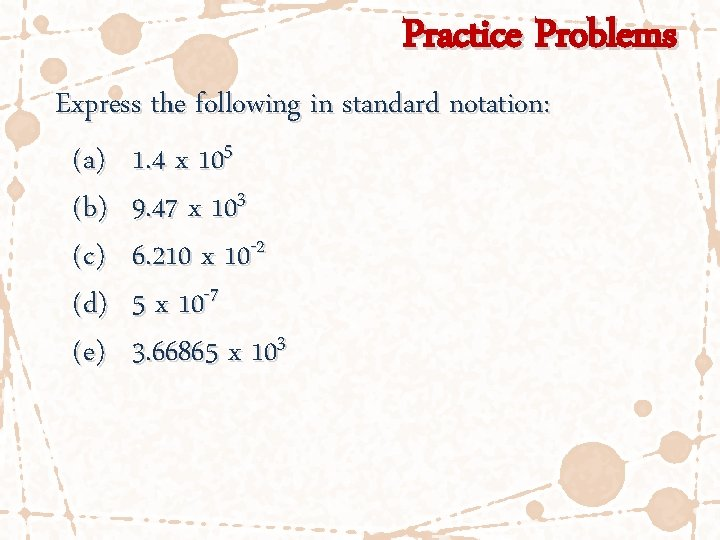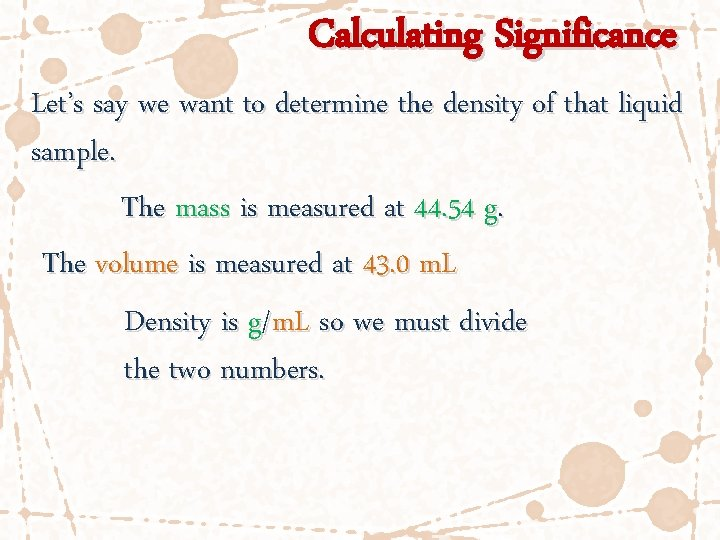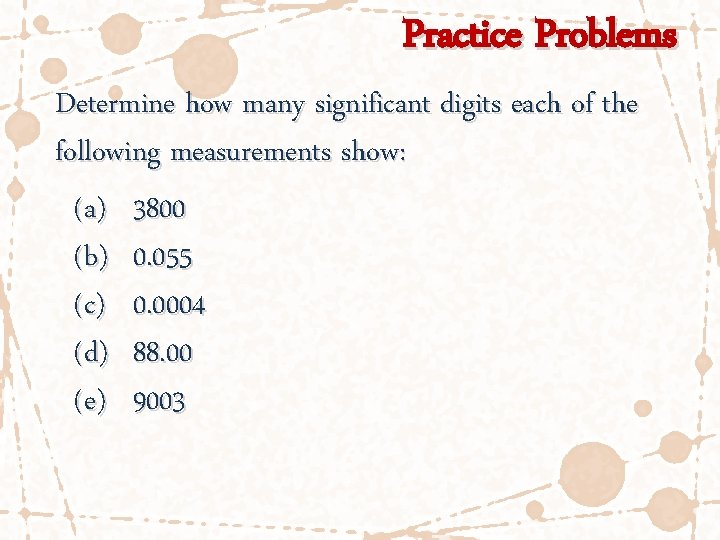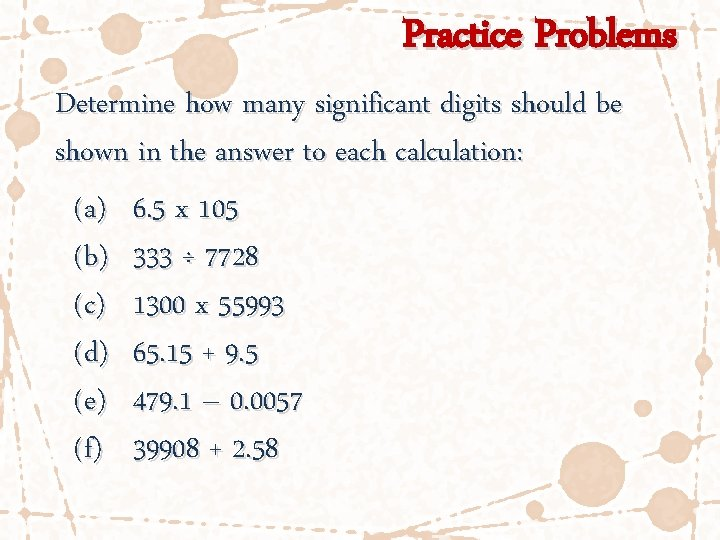# Significant Digits and Scientific Notation Scientific Notation In

• Slides: 13Significant Digits and Scientific NotationScientific Notation In chemistry you will be using data that are measured in very small and very large scales. Scientific Notation allows us to express these very large or small numbers in simpler ways. We express numbers with a single units digit, additional digits behind the decimal, then multiply by 10 raised to a power to show the scale of the number.Scientific Notation 1748590 would be expressed as: 1. 748590 x 106 0. 0006743 would be expressed as: 6. 743 x 10 -4 For decimal shifting and exponent changes remember the acronym: LIRD shifting the decimal left, increases the exponent shifting the decimal right, decreases the exponentPractice Problems Express the following in scientific notation: (a) 45600 (b) 134 (c) 0. 0095 (d) 9500000 (e) 0. 000531Practice Problems Express the following in standard notation: (a) 1. 4 x 105 (b) 9. 47 x 103 (c) 6. 210 x 10 -2 (d) 5 x 10 -7 (e) 3. 66865 x 103Significance of Measurements The number of digits shown in a measurement is limited by the scale of the measuring device. The graduated cylinder is precise to the ones digit. We record a measurement to the tenths (0. 1) digit. (one estimated digit)Significance of Measurements The volume measurement would be 43. 0 m. L. Each digit communicates scale and is significant. 40 m. L would be too vague. (tools are more specific) 43. 00184 m. L would be too specific. (tools are not this precise)Calculating Significance Let’s say we want to determine the density of that liquid sample. The mass is measured at 44. 54 g. The volume is measured at 43. 0 m. L Density is g/m. L so we must divide the two numbers.Calculating Significance The mass is measured at 44. 54 g. The volume is measured at 43. 0 m. L 44. 54 g ÷ 43. 0 m. L = 1. 03581… g/m. L Our answer is limited to have the same number as the LEAST precise measurement. Our final answer must be rounded to 1. 04 g/m. L only 3 digits.Sig Fig Rules 1) All non-zero digits are always significant. 595 2) Zeroes between non-zero digits are always significant. 505 3) For numbers 1, zeroes at the end are never significant unless followed by a decimal point. 500.Sig Fig Rules 4) For numbers 1, zeroes before a non-zero are never significant. 0. 00505 5) All digits following a non-zero in a number with a decimal are always significant. 505. 00Practice Problems Determine how many significant digits each of the following measurements show: (a) 3800 (b) 0. 055 (c) 0. 0004 (d) 88. 00 (e) 9003Practice Problems Determine how many significant digits should be shown in the answer to each calculation: (a) 6. 5 x 105 (b) 333 ÷ 7728 (c) 1300 x 55993 (d) 65. 15 + 9. 5 (e) 479. 1 – 0. 0057 (f) 39908 + 2. 58